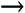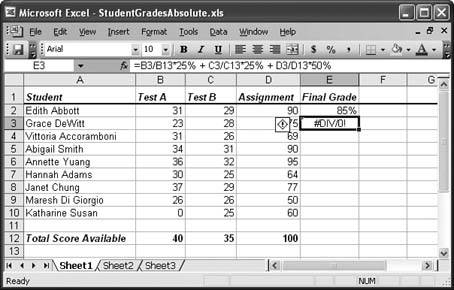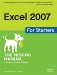Section 7.3. Copying Formulas

7.3. Copying Formulas

Sometimes you need to perform similar calculations in different cells throughout a worksheet. For example, you may want to calculate sales tax on each item in a product catalog, the monthly sales in each store of a company, or the final grade for each student in a class. In this section, you'll learn how Excel makes doing these kinds of calculations easy with relative cell references. Relative cell references are cell references that Excel updates automatically when you copy them from one cell into another. They're the standard kind of references that Excel uses (as opposed to absolute cell references, which are covered in the next section). In fact, all the references you've used so far have been relative references, but you haven't yet seen how they work with copy-and-paste operations.

Take a look at the worksheet shown in Figure 7-8, which contains a teacher's grade book. In this example, each student has three grades: two tests and one assignment. You want to base each student's final grade on the following percentages: 25 percent for each of the two tests, and 50 percent for the assignment.

The following formula calculates the final grade for the first student (Edith Abbott):

=B2*25% + C2*25% + D2*50%

The formula that calculates the final grade for the second student (Grace DeWitt) is almost identical. The only change is that all the cell references are offset by one row, so that B2 becomes B3, C2 becomes C3, and D2 becomes D3:

=B3*25% + C3*25% + D3*50%

The formula that calculates the final grade for the third student (Vittoria Accoramboni), once again, is almost identical. Once again, the cell references are offset by one row:

=B4*25% + C4*25% + D4*50%

And so on.

Figure 7-8. This worksheet shows a list of students in a class, and calculates the final grade for each using two test scores and an assignment score. So far, the only formula that's been added is for the first student (in cell E2).Entering all these formulas by hand is painstaking. A far easier approach: copy the formula from one cell to another. Here's how:

1. Move to the cell containing the formula you want to copy.

In this example, you'd move to cell E2.

2. Copy the formula to the clipboard by selecting EditCopy.

Select the range of cells you want to copy the formula into.

Select cells E3 to E10.

3. Paste in the new formulas by selecting EditPaste (Ctrl+V).

Figure 7-9. When you paste the formula from Cell E2 into a range of cells (E3:E10), each Final Grade formula operates on the data in its own row. This means that you don't have to tweak the formula for each student. Copy, pasteyou're good to go.Tip: The AutoFill feature introduced in Chapter 2 (Section 2.2.3) offers an even quicker way to copy a formula to multiple cells. In the student grade example, you start by moving to cell E2, which contains the original formula. Then, you click the small square at the bottom-right corner of the cell outline, and drag the outline down until it covers all cells from E3 to E10. When you release the mouse button, Excel inserts formula copies into each and every cell in the AutoFill region.

7.3.1. Absolute Cell References

Relative references are a true convenience in that they let you create formula copies that don't need the slightest bit of editing. But you've probably already realized that relative references don't always work. For example, what if you have a value in a specific cell that you want to use in multiple calculations?

Say you have a currency conversion ratio that you want to use in a list of expenses. Each item in the list needs to use the same specific cell to perform the conversion correctly. But if you make copies of the formula using relative cell references, Excel adjusts this reference automatically, and the formula ends up referring to the wrong cell (and therefore the wrong conversion value).

Figure 7-10 illustrates the problem with the worksheet of student grades. In this example, the test and assignment scores aren't all graded based on 100 possible points; instead, each item has a different total score available (listed in row 12). So in order to calculate the percentage a student earned on a test, you need to divide each student's test score by the total score available. This formula, for example, calculates the percentage for Edith Abbott's performance on Test A:

=B2/B12*100%

To calculate Edith's final grade for the class, you'd use the following formula:

=B2/B12*25% + C2/C12*25% + D2/D12*50%

Like many formulas, this one contains a mix of cells that should be relative (the individual scores in cells B2, C2, and D2) and those that should be absolute (the possible totals in cell B12, C12, and D12). As you copy this formula to subsequent rows, Excel incorrectly changes all the cell references, causing a calculation error.

Fortunately, Excel provides a perfect solution. It lets you use absolute cell referencescell references that always refer to the same cell. When you create a copy of a formula that contains an absolute cell reference, Excel doesn't change the reference (as it does when you use relative cell references; see the previous section). To indicate that a cell reference is absolute, use the dollar sign (\$) character. For example, to change B12 into an absolute reference, you add the \$ character twice: once before the column letter, and once before the row number, like this: \$B\$12.

Figure 7-10. Here, both the tests and the assignment are graded on different scales (as listed in row 12). So the formula for calculating the final class grade uses the values in cells B12, C12, and D12. When you copy Cell E to the rows below it, Excel offsets the formula to use B13, C13, and D13none of which exist. Oops! That's a problemindicated here by a divide-by-zero error. To fix this error, use absolute cell references.Here's the corrected final grade formula (for Edith) using absolute cell references:

=B2/\$B\$12*25% + C2/\$C\$12*25% + D2/\$D\$12*50%

This formula still produces the same result for the first student (Edith). But now you can copy it into all the cells in column E and have it work correctly for the other students. To copy this formula into all the cells in column E, use the same procedure described in the previous section on relative cell references.

UP TO SPEED
Creating an Exact Formula Copy

Excel lets you copy a formula (without automatically changing the formula's cell references) one other way: by copying the formula itself, rather than copying the whole cell (which is what you do when performing a basic copy-and-paste operation on a formula).

The process takes a few more steps, and it lets you paste only one copy at a time, but it can still come in handy if you don't want Excel to use relative references. Here's how it works:

1. First, move to the cell that contains the formula you want to copy.

2. Place this cell in edit mode by double-clicking it or pressing F2.

3. Select all the text in the cell. You can use the mouse, or you can use the arrow keys (just hold down Shift as you scroll from the beginning to the end of the cell).

4. Once you've selected the complete formula, press Ctrl+C to copy it (or select EditCopy).

5. Move to the new cell, and press Ctrl+V to paste it (or select EditPaste from the menu).

7.3.2. Partially Fixed References

You may wonder why you need to use the \$ character twice in an absolute reference (before the column letter and the row number). The reason is because Excel lets you create partially fixed references. To understand partially fixed references, it helps to remember that every cell reference consists of a column letter and a row number. With a partially fixed reference, Excel updates one component (say, the column part) but not the other (the row) when you copy the formula. If this sounds complex (or a little bizarre), take a look at a few scenarios where partially fixed references make sense:

• You have a loan rate in cell A1, and you want all loans on an entire worksheet to use that rate in calculations. If you refer to the cell as \$A\$1, its column and row always stay the same when you copy the formula to another cell. Absolute cell referencing works perfectly in this scenario.

 FREQUENTLY ASKED QUESTIONHow Changing the Location of Cells Affects Formulas OK, I know how Excel adjusts a formula when I copy it to another location. But what happens if I move cells around after I've created a formula?No worries. It turns out that Excel is surprisingly intelligent. Take a look at the following simple formula: =B1+A2 If you cut and paste the contents of A2 to A3, Excel automatically updates your formula to point to the new cell, without complaining once. It also performs the same automatic cleanup if you drag the contents of a cell to another location (although if you simply make a duplicate copy of the cell, Excel doesn't change your formula).Excel is also on the ball when you insert and delete rows and columns.If at any time Excel can't find your cells, it changes the formula to show the error code #REF! You can then take a closer look at the formula (using the Formula bar) to find out what really went wrong. For example, if you delete column B from your spreadsheet (by selecting the column and using the EditDelete command), the formula changes to this: =#REF!+A2 Even though a B1 cell still exists in your worksheet (it's the cell that was formerly named C1), Excel modifies the formula to make it clear that you've lost your original data.

• You have several rows of loan information. The first column of a row always contains the loan rate for all loans on that row. In your formula cell, if you refer to cell \$A1, when you copy the formula across columns and rows, the row changes (2, 3, 4, and so on) but the column doesn't (A2, A3, A4, and so on). Partially fixed cell referencingfixing just the column reference, not the row referencedoes the job.

• You have a table of loan rates organized by the length of the loan (10-year, 15-year, 20-year, etc.) along the top of a worksheet. Loans in each column are calculated using the rate specified at the top of that column. If you refer to the rate cell as A\$1 in your first column's formula, the row stays constant (1), but the column changes (B1, C1, D1, and so on) as you copy the formula across columns and down rows. Again, partially fixed cell referencing is perfect for the job: but this time, it's the row reference you fix, not the column reference.

Tip: You can quickly change formula references into absolute or partially fixed references. Just put the cell into edit mode (by double-clicking it or pressing F2). Then, move through the formula until you've highlighted the appropriate cell reference. Now, press F4 to change the cell reference. Each time you press F4, the reference changes. If the reference is A1, for instance, it becomes \$A\$1, then A\$1, then \$A1, and then A1 again.Excel 2007 for Starters: The Missing Manual
ISBN: 0596528329
EAN: 2147483647
Year: 2003
Pages: 85

Similar book on Amazon